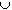#Interactive Real Analysis

Next | Previous | Glossary | Map

## 1.1. Notation and Set Theory

### Definition 1.1.1: Sets and Operations on Sets

A set is a collection of objects chosen from some universe. The universe is usually understood from the context. Sets are denoted by capital letters or curly brackets.
• AB: A is a subset of B means that every element in A is also contained in B.
• AB: A union B is the set of all elements that are either in A or in B or in both sets.
• AB: A intersection B is the set of all elements that are in both sets A and B.
• A \ B: A minus B are all elements from A that are not in B.
• comp(A): The complement of A consists of all elements that are not in A.
• Two sets are disjoint if AB = 0 (the empty set)
• Two sets A and B are equal if AB and BA
Next | Previous | Glossary | Map